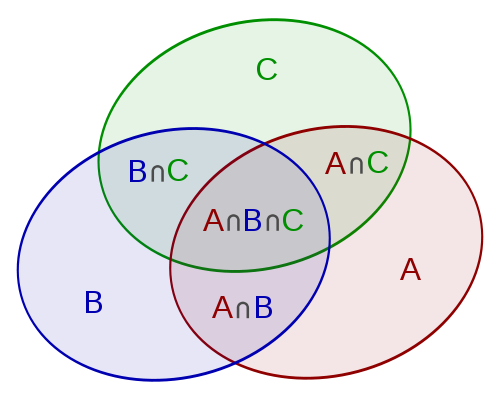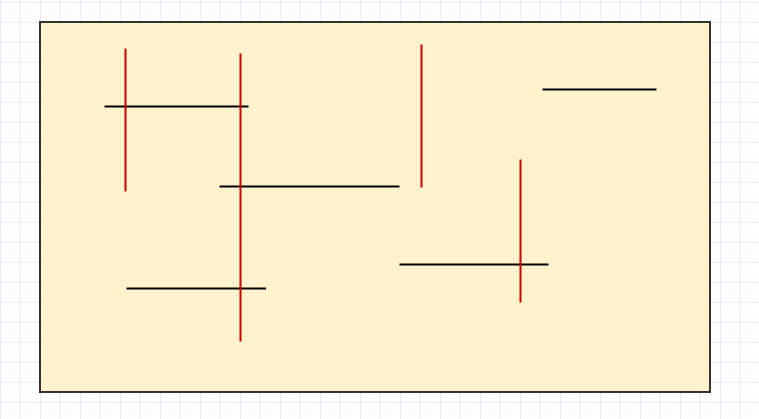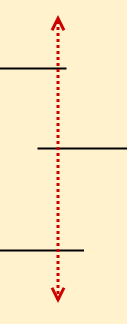## 什么是容斥原理## 容斥原理的应用

### 考虑一个问题

1. N以内的，能被2整除的数
2. N以内的，能被3整除的数

## 一种区间交问题的奇怪姿势

Update: 原来这种数据结构叫珂朵莉树啊，真神奇。。。

## A. 2月29日 (Feb. 29th)

### Description

Given a starting date and an ending date. Count how many Feb. 29th are between the given dates.

### Solution

The easiest way, of course, the brute force, which is quite simple with Python using the `datetime` lib.

However, it's not an effective way for the …

100组数据。

## A. Fox And Snake

Implementation

```(n, m) = map(int, raw_input().split())

res = []

for i in xrange(n):
if i % 2 == 0:
res.append('#' * m)
elif (i / 2) % 2 == 0:
res.append('.' * (m - 1) + '#')
else:
res.append('#' + '.' * (m - 1))

for line in res:
print line
```

DFS …

## A. Maximum in Table

Simulation.

```n = int(raw_input())
g = [[1 for i in xrange(n)] for j in xrange(n)]

for i in xrange(1, n):
for j in xrange(1, n):
g[i][j] = g[i - 1][j] + g[i][j - 1]

print g[n - 1][n - 1]
```

## A. Pasha and Pixels

Brute force.

There are multiple ways to form a 2*2 square at one single step.So at every step, we have to check the neighbours of pixel that is colored black.

```#include <cstdio>
#include <cstdlib>
#include <cstring>
#include <iostream>
#include <vector>

using namespace std;

#define …```

## 题目## 分析